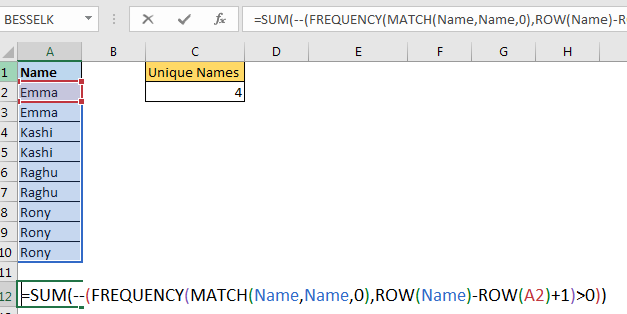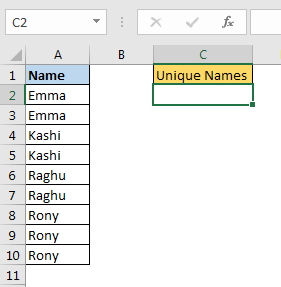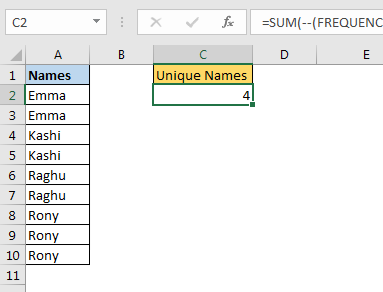# How To Count Unique Text in Excel

Well we have counted unique values using COUNTIF and SUMPRODUCT function. Although that method is easy but that is slow when data is large. In this article, we will learn how to count unique text values in excel with a faster formulaGeneric formula to count unique text values in excel

=SUM(--(FREQUENCY(MATCH(range,range,0),ROW(first_cell_in_range)+1)>0))

Range : The range from which you want to get unique values.

firstCell in range: It is the reference of the first cell in range. If range is A2:A10 then it is A2.

Let’s see an example to make things clear.

Example: Count Unique Text Values Excel
In an excel sheet, I have this data of names in range A2:A10. I want to get count of unique names from the given range.Apply above generic formula here to count unique text in excel range A2:A10. I have named A2:A10 as names.

=SUM(--(FREQUENCY(MATCH(names,names,0),ROW(A2)+1)>0))

This returns the total count of unique texts in range A2:A10.How it works?
Let’s solve it from inside.

MATCH(names,names,0): this part will return the first location of each value in range A2:A10 (names) as per MATCH’s property.

{1;1;3;3;5;5;7;7;7}.

Next ROW(A2:A19): This returns the row number of each cell in range A2:A10.

{2;3;4;5;6;7;8;9;10}

ROW(names)-ROW(A2): Now we subtract the first row number from each row number. This returns the an array of serial number starting from 0.

{0;1;2;3;4;5;6;7;8}

Since we want to have serial number starting from 1, we add 1 to it.

ROW(names)-ROW(A2)+1. This gives us an array of serial number starting from 1.

{1;2;3;4;5;6;7;8;9}

This will help us in getting unique count on condition.
Now we have:

FREQUENCY({1;1;3;3;5;5;7;7;7},{1;2;3;4;5;6;7;8;9}).

This returns the frequency of each number in given array.{2;0;2;0;2;0;3;0;0;0}

Here each positive number indicated occurrence of unique value when criteria is met. We need to count values greater than 0 in this array. For that we check it by >0. This will return TRUE and FALSE. We convert true false using -- (double binary operator).

SUM(--({2;0;2;0;2;0;3;0;0;0})>0) this translates toSUM({1;0;1;0;1;0;1;0;0;0})

And finally we get the unique count of names in range on criteria as 4.

How to count unique text in range with blank cells?

The problem with above formula is that when you have blank cell in range, it will pop #N/A error. To tackle this we need to put a condition to check blank cells.

=SUM(--(FREQUENCY(IF(names <> “”,MATCH(names,names,0)),ROW(A2)+1)>0))

This will give correct output. Here we have encapsulated MATCH with IF function. You can read the full explanation in article How To Count Unique Values in Excel With Multiple Criteria?

So yeah guys, this how you can get unique text count in excel. Let me know if you have any doubts regarding this or any other advance excel/vba topic. The comments section is open for you.

Related Articles:

How To Count Unique Values in Excel With Criteria

Excel Formula to Extract Unique Values From a List

Count Unique Values In Excel
Popular Articles:

The VLOOKUP Function in Excel

COUNTIF in Excel 2016

How to Use SUMIF Function in Excel

Terms and Conditions of use

The applications/code on this site are distributed as is and without warranties or liability. In no event shall the owner of the copyrights, or the authors of the applications/code be liable for any loss of profit, any problems or any damage resulting from the use or evaluation of the applications/code.